# MP Board Class 10th Maths Solutions Chapter 3 Pair of Linear Equations in Two Variables Ex 3.3

In this article, we will share MP Board Class 10th Maths Book Solutions Pair of Linear Equations in Two Variables Ex 3.3 Pdf, These solutions are solved subject experts from the latest edition books.

## MP Board Class 10th Maths Solutions Chapter 3 Pair of Linear Equations in Two Variables Ex 3.3

Question 1.
Solve the following pair of linear equations by the substitution method.
(i) x + y = 14; x – y = 4
(ii) s – f = 3; $$\frac{s}{3}+\frac{t}{2}$$ = 6
(iii) 3x – y = 3; 9x – 3y = 9
(iv) 0.2x + 0.3y = 1.3; 0.4x + 0.5y = 2.3
(v) $$\sqrt{2} x+\sqrt{3} y$$ = 0; $$\sqrt{3} x-\sqrt{8} y$$ = 0
(vi) $$\frac{3 x}{2}-\frac{5 y}{3}$$ = -2, $$\frac{x}{3}+\frac{y}{2}=\frac{13}{6}$$
Solution:
(i) x + y = 14 … (1),
x – y = 4 …. (2)
From (1) , we get x = (14 – y) …. (3)
Substituting value of x in (2) , we get
(14 – y) – y = 4 ⇒ 14 – 2y = 4 ⇒ -2y = -10 ⇒ y = 5
Substituting y = 5 in (3), we have
x = 14 – 5 ⇒ x = 9
Hence, x = 9, y = 5

(ii) s – t = 3 … (1)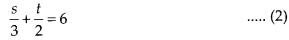From (1), we have s = (3 + t) … (2)
Substituting this value of s in (2), we get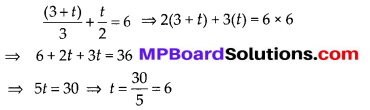Substituting, t = 6 in (3) we get,
S = 3 + 6 = 9
Thus, S = 9, f = 6

(iii) 3x – y = 3 … (1),
9x – 3y = 9 … (2)
From (1) , y = (3x – 3)
Substituting this value of y in (2),
9x – 3(3x – 3) = 9
⇒ 9x – 9x + 9 = 9 ⇒ 9 = 9 which is true,
Eq. (1) and eq. (2) have infinitely many solutions.

(iv) 0.2x + 0.3y = 1.3 … (1)
0.4x + 0.5y = 2.3 …. (2)
From the equation (1),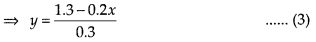Substituting the value of y in (2), we have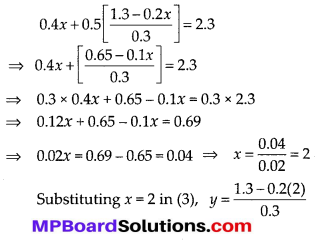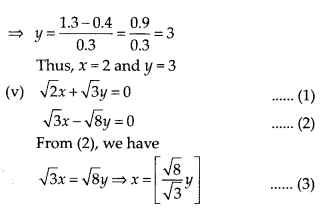Substituting the value of x in (1), we have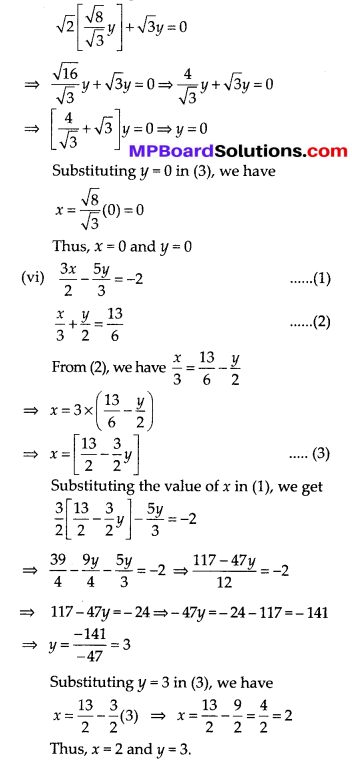Question 2.
Solve 2x + 3y = 11 and 2x – 4y = -24 and hence find the value of ‘m’ for which y = mx + c
Solution: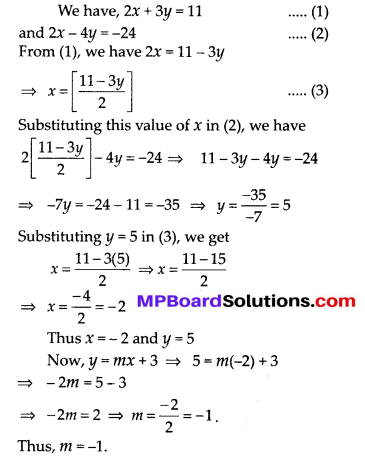Question 3.
Form the pair of linear equations for the following problems and find their solution by
substitution method.
(i) The difference between two numbers is 26 and one number is three times the other. Find them.
(ii) The larger of two supplementary angles exceeds the smaller by 18 degrees. Find them.
(iii) The coach of a cricket team buys 7 bats and 6 balls for 13800. Later, she buys 3 bats and 5 balls for ₹ 1750. Find the cost of each bat and each ball.
(iv) The taxi charges in a city consist of a fixed charge together with the charge for the distance covered. For a distance of 10 km, the charge paid is ₹ 105 and for a journey of 15 km, the charge paid is ₹ 155. What are the fixed charges and the charge per km? How much does a person have to pay for travelling a distance of 25 km?
(v) A fraction becomes 9/11, if 2 is added to both the numerator and the denominator. If, 3 is added to both the numerator and the denominator it becomes 5/6. Find the fraction.
(vi) Five years hence, the age of Jacob will be three times that of his son. Five years ago, Jacob’s age was seven times that of his son. What are their present ages?
Solution:
(i) Let the two numbers be X and y such that x > y
It is given that
Difference between two numbers = 26
∴ x – y = 26 … (1)
Also one number = 3 [the other number]
⇒ x = 3y … (2)
Substituting x = 3y in (1) , we get 3y – y = 26 ⇒ 2y = 26
Now, substituting y = 13 in (2) , we have
x = 3(13) ⇒ x = 39
Thus, two numbers are 39 and 13.

(ii) Let the two angles be x and y such that x > y
∵ The larger angle exceeds the smaller by 18° (Given)
∴ x = y + 18°…. (1)
Also, sum of two supplementary angles = 180°
∴ x + y = 180° … (2)
Substituting the value of x from (1) in (2) , we get,
(18° + y) + y = 180°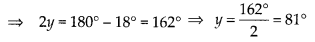Substituting, y = 81° in (1) , we get
x = 18° + 81° = 99°
Thus, x = 99° and y = 81°

(iii) Let the cost of a bat = ₹ x
And the cost of a ball = ₹ y
∵ [cost of 7 bats] + [cost of 6 balls] = ₹ 3800
⇒ 7x + 6y = 3800 … (1)
Also, [cost of 3 bats] + [cost of 5 balls] = ₹ 1750
3x + 5y = 1750 …. (2)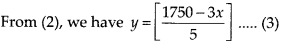Substituting this value of y in (1) , we have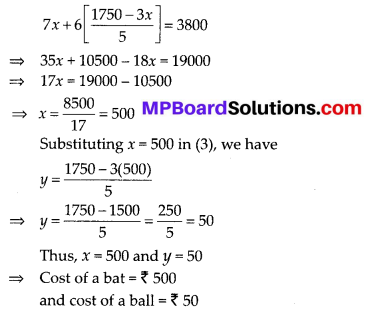(iv) Let fixed charges = ₹ x
and charges per km = ₹ y
∵ Charges for the journey of 10 km = ₹ 105 (Given)
∴ x + 10y = 105 … (1)
and charges for the journey of 15 km = ₹ 155
∴ x + 15y = 155 … (2)
From (1) , we have, x = 105 – 10y …. (3)
Putting the value of x in (2) , we get
(105 – 10y) + 15y = 155
⇒ 5y = 155 – 105 = 50 ⇒ y = 10
Substituting y = 10 in (3) , we get
x = 105 – 10(10) ⇒ x = 105 – 100 = 5
Thus, x = 5 and y = 10
⇒ Fixed charges = ₹ 5
and charges per km = ₹ 10
Now, charges for 25 km = x + 25y = 5 + 25(10) = 5 + 250 = ₹ 255
∴ The charges for 25 km journey = ₹ 255(v) Let the numerator = x
and the denominator = y
∴ Fraction = $$\frac{x}{y}$$
According to the given condition,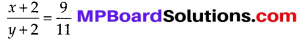⇒ 11(x + 2) = 9(y + 2)
⇒ 11x + 22 = 9y + 18
⇒ 11x – 9y + 4 = 0 … (1)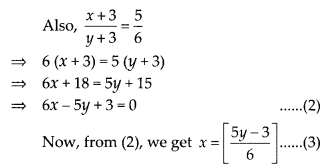Substituting this value of x in (1) , we have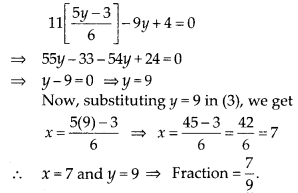(vi) Let the present age of Jacob = x years
and the present age of his son = y years
∴ 5 years hence: Age of Jacob = (x + 5) years
Age of his son = (y + 5) years
According to given condition,
[Age of Jacob] = 3[Age of his son]
x + 5 = 3(y + 5) ⇒ x + 5 = 3y + 15
⇒ x – 3y -10 = 0 … (1)
5years ago : Age of Jacob = (x – 5) years,
Age of his son = (y – 5) years
According to given condition,
[Age of Jacob] = 7[Age of his son]
∴ (x – 5) = 7(y – 5) ⇒ x – 5 = 7y – 35
⇒ x – 7y + 30 = 0 … (2)
From (1) , x = [10 + 3y] … (3)
Substituting this value of x in (2) , we get
(10 + 3y) – 7y + 30 = 0
⇒ -4y = -40 ⇒ y = 10
Now, substituting y = 10 in (3) ,
we get x = 10 + 3(10)
⇒ x = 10 + 30 = 40
Thus, x = 40 and y = 10
⇒ Present age of Jacob = 40 years and present age of his son = 10 years